# Basics of estimated marginal means

## Why we need EMMs

Consider the pigs dataset provided with the package (help("pigs") provides details). These data come from an unbalanced experiment where pigs are given different percentages of protein (percent) from different sources (source) in their diet, and later we measure the concentration (conc) of leucine. Here’s an interaction plot showing the mean conc at each combination of the other factors.

with(pigs, interaction.plot(percent, source, conc))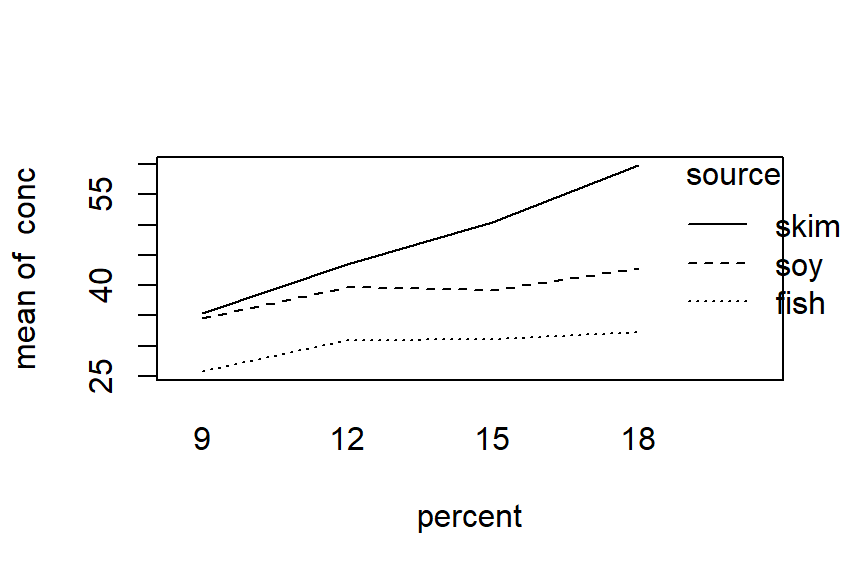This plot suggests that with each source, conc tends to go up with percent, but that the mean differs with each source.

Now, suppose that we want to assess, numerically, the marginal results for percent. The natural thing to do is to obtain the marginal means:

with(pigs, tapply(conc, percent, mean))
##        9       12       15       18
## 32.70000 38.01111 40.12857 39.94000

Looking at the plot, it seems a bit surprising that the last three means are all about the same, with the one for 15 percent being the largest.

Hmmmm, so let’s try another approach – actually averaging together the values we see in the plot. First, we need the means that are shown there:

cell.means <- matrix(with(pigs,
tapply(conc, interaction(source, percent), mean)),
nrow = 3)
cell.means
##          [,1]     [,2]     [,3]     [,4]
## [1,] 25.75000 30.93333 31.15000 32.33333
## [2,] 34.63333 39.63333 39.23333 42.90000
## [3,] 35.40000 43.46667 50.45000 59.80000

Confirm that the rows of this matrix match the plotted values for fish, soy, and skim, respectively. Now, average each column:

apply(cell.means, 2, mean)
##  31.92778 38.01111 40.27778 45.01111

These results are decidedly different from the ordinary marginal means we obtained earlier. What’s going on? The answer is that some observations were lost, making the data unbalanced:

with(pigs, table(source, percent))
##       percent
## source 9 12 15 18
##   fish 2  3  2  3
##   soy  3  3  3  1
##   skim 3  3  2  1

We can reproduce the marginal means by weighting the cell means with these frequencies. For example, in the last column:

sum(c(3, 1, 1) * cell.means[, 4]) / 5
##  39.94

The big discrepancy between the ordinary mean for percent = 18 and the marginal mean from cell.means is due to the fact that the lowest value receives 3 times the weight as the other two values.

### The point

The point is that the marginal means of cell.means give equal weight to each cell. In many situations (especially with experimental data), that is a much fairer way to compute marginal means, in that they are not biased by imbalances in the data. We are, in a sense, estimating what the marginal means would be, had the experiment been balanced. Estimated marginal means (EMMs) serve that need.

All this said, there are certainly situations where equal weighting is not appropriate. Suppose, for example, we have data on sales of a product given different packaging and features. The data could be unbalanced because customers are more attracted to some combinations than others. If our goal is to understand scientifically what packaging and features are inherently more profitable, then equally weighted EMMs may be appropriate; but if our goal is to predict or maximize profit, the ordinary marginal means provide better estimates of what we can expect in the marketplace.

Back to Contents

## What exactly are EMMs?

### Model and reference grid

Estimated marginal means are based on a model – not directly on data. The basis for them is what we call the reference grid for a given model. To obtain the reference grid, consider all the predictors in the model. Here are the default rules for constructing the reference grid

• For each predictor that is a factor, use its levels (dropping unused ones)
• For each numeric predictor (covariate), use its average.1

The reference grid is then a regular grid of all combinations of these reference levels.

As a simple example, consider again the pigs dataset (see help("fiber") for details). Examination of residual plots from preliminary models suggests that it is a good idea to work in terms of log concentration.

If we treat the predictor percent as a factor, we might fit the following model:

pigs.lm1 <- lm(log(conc) ~ source + factor(percent), data = pigs)

The reference grid for this model can be found via the ref_grid function:

ref_grid(pigs.lm1)
## 'emmGrid' object with variables:
##     source = fish, soy, skim
##     percent =  9, 12, 15, 18
## Transformation: "log"

Both predictors are factors, and the reference grid consists of the $$3\times4 = 12$$ combinations of these factor levels. It can be seen explicitly by looking at the grid slot of this object:

ref_grid(pigs.lm1) @ grid
##    source percent .wgt.
## 1    fish       9     2
## 2     soy       9     3
## 3    skim       9     3
## 4    fish      12     3
## 5     soy      12     3
## 6    skim      12     3
## 7    fish      15     2
## 8     soy      15     3
## 9    skim      15     2
## 10   fish      18     3
## 11    soy      18     1
## 12   skim      18     1

Note that other information is retained in the reference grid, e.g., the transformation used on the response, and the cell counts as the .wgt. column.

Now, suppose instead that we treat percent as a numeric predictor. This leads to a different model – and a different reference grid.

pigs.lm2 <- lm(log(conc) ~ source + percent, data = pigs)
ref_grid(pigs.lm2)
## 'emmGrid' object with variables:
##     source = fish, soy, skim
##     percent = 12.931
## Transformation: "log"

This reference grid has the levels of source, but only one percent value, its average. Thus, the grid has only three elements:

ref_grid(pigs.lm2) @ grid
##   source  percent .wgt.
## 1   fish 12.93103    10
## 2    soy 12.93103    10
## 3   skim 12.93103     9

Back to Contents

### Estimated marginal means

Once the reference grid is established, we can consider using the model to estimate the mean at each point in the reference grid. (Curiously, the convention is to call this “prediction” rather than “estimation”). For pigs.lm1, we have

pigs.pred1 <- matrix(predict(ref_grid(pigs.lm1)), nrow = 3)
pigs.pred1
##          [,1]     [,2]     [,3]     [,4]
## [1,] 3.220292 3.399846 3.437691 3.520141
## [2,] 3.493060 3.672614 3.710459 3.792909
## [3,] 3.622569 3.802124 3.839968 3.922419

Estimated marginal means (EMMs) are defined as equally weighted means of these predictions at specified margins:

apply(pigs.pred1, 1, mean) ### EMMs for source
##  3.394492 3.667260 3.796770
apply(pigs.pred1, 2, mean) ### EMMs for percent
##  3.445307 3.624861 3.662706 3.745156

For the other model, pigs.lm2, we have only one point in the reference grid for each source level; so the EMMs for source are just the predictions themselves:

predict(ref_grid(pigs.lm2))
##  3.379865 3.652693 3.783120

These are slightly different from the previous EMMs for source, emphasizing the fact that EMMs are model-dependent. In models with covariates, EMMs are often called adjusted means.

The emmeans function computes EMMs, accompanied by standard errors and confidence intervals. For example,

emmeans(pigs.lm1, "percent")
##  percent emmean     SE df lower.CL upper.CL
##        9   3.45 0.0409 23     3.36     3.53
##       12   3.62 0.0384 23     3.55     3.70
##       15   3.66 0.0437 23     3.57     3.75
##       18   3.75 0.0530 23     3.64     3.85
##
## Results are averaged over the levels of: source
## Results are given on the log (not the response) scale.
## Confidence level used: 0.95

In these examples, all the results are presented on the log(conc) scale (and the annotations in the output warn of this). It is possible to convert them back to the conc scale by back-transforming. This topic is discussed in the vignette on transformations.

An additional note: There is an exception to the definition of EMMs given here. If the model has a nested structure in the fixed effects, then averaging is performed separately in each nesting group. See the section on nesting in the “messy-data” vignette for an example.

Back to Contents

### Altering the reference grid

It is possible to alter the reference grid. We might, for example, want to define a reference grid for pigs.lm2 that is comparable to the one for pigs.lm1.

ref_grid(pigs.lm2, cov.keep = "percent")
## 'emmGrid' object with variables:
##     source = fish, soy, skim
##     percent =  9, 12, 15, 18
## Transformation: "log"

Using cov.keep = "percent" specifies that, instead of using the mean, the reference grid should use all the unique values of each covariate“percent”.

Another option is to specify a cov.reduce function that is used in place of the mean; e.g.,

ref_grid(pigs.lm2, cov.reduce = range)
## 'emmGrid' object with variables:
##     source = fish, soy, skim
##     percent =  9, 18
## Transformation: "log"

Another option is to use the at argument. Consider this model for the built-in mtcars dataset:

mtcars.lm <- lm(mpg ~ disp * cyl, data = mtcars)
ref_grid(mtcars.lm)
## 'emmGrid' object with variables:
##     disp = 230.72
##     cyl = 6.1875

Since both predictors are numeric, the default reference grid has only one point. For purposes of describing the fitted model, you might want to obtain predictions at a grid of points, like this:

mtcars.rg <- ref_grid(mtcars.lm, cov.keep = 3,
at = list(disp = c(100, 200, 300)))
mtcars.rg
## 'emmGrid' object with variables:
##     disp = 100, 200, 300
##     cyl = 4, 6, 8

This illustrates two things: a new use of cov.keep and the at argument. cov.keep = "3" specifies that any covariates having 3 or fewer unique values is treated like a factor (the system default is cov.keep = "2"). The at specification gives three values of disp, overriding the default behavior to use the mean of disp. Another use of at is to focus on only some of the levels of a factor. Note that at does not need to specify every predictor; those not mentioned in at are handled by cov.reduce, cov.keep, or the default methods. Also, covariate values in at need not be values that actually occur in the data, whereas cov.keep will use only values that are achieved.

Back to Contents

### Derived covariates

You need to be careful when one covariate depends on the value of another. To illustrate in the mtcars example, suppose we want to use cyl as a factor and include a quadratic term for disp:

mtcars.1 <- lm(mpg ~ factor(cyl) + disp + I(disp^2), data = mtcars)
emmeans(mtcars.1, "cyl")
##  cyl emmean   SE df lower.CL upper.CL
##    4   19.3 2.66 27     13.9     24.8
##    6   17.2 1.36 27     14.4     20.0
##    8   18.8 1.47 27     15.7     21.8
##
## Confidence level used: 0.95

Some users may not like function calls in the model formula, so they instead do something like this:

mtcars <- transform(mtcars,
Cyl = factor(cyl),
dispsq = disp^2)
mtcars.2 <- lm(mpg ~ Cyl + disp + dispsq, data = mtcars)
emmeans(mtcars.2, "Cyl")
##  Cyl emmean   SE df lower.CL upper.CL
##  4     20.8 2.05 27     16.6     25.0
##  6     18.7 1.19 27     16.3     21.1
##  8     20.2 1.77 27     16.6     23.9
##
## Confidence level used: 0.95

Wow! Those are really different results – even though the models are equivalent. Why is this? To understand, look at the reference grids:

ref_grid(mtcars.1)
## 'emmGrid' object with variables:
##     cyl = 4, 6, 8
##     disp = 230.72
ref_grid(mtcars.2)
## 'emmGrid' object with variables:
##     Cyl = 4, 6, 8
##     disp = 230.72
##     dispsq = 68113

For both models, the reference grid uses the disp mean of 230.72. But for mtcars.2, we also set dispsq to its mean of 68113. This is not right, because dispsq should be the square of disp (about 53232, not 68113) in order to be consistent. If we use that value of dispsq, we get the same results (modulus rounding error) as for mtcars.1:

emmeans(mtcars.2, "Cyl", at = list(dispsq = 230.72^2))
##  Cyl emmean   SE df lower.CL upper.CL
##  4     19.3 2.66 27     13.9     24.8
##  6     17.2 1.36 27     14.4     20.0
##  8     18.8 1.47 27     15.7     21.8
##
## Confidence level used: 0.95

In summary, for polynomial models and others where some covariates depend on others in nonlinear ways, include that dependence in the model formula (as in mtcars.1) using I() or poly() expressions, or alter the reference grid so that the dependency among covariates is correct.

### Non-predictor variables

Reference grids are derived using the variables in the right-hand side of the model formula. But sometimes, these variables are not actually predictors. For example:

deg <- 2
mod <- lm(y ~ treat * poly(x, degree = deg), data = mydata)

If we call ref_grid() or emmeans() with this model, it will try to construct a grid of values of treat, x, and deg – causing an error because deg is not a predictor in this model. To get things to work correctly, you need to name deg in a params argument, e.g.,

emmeans(mod, ~ treat | x, at = list(x = 1:3), params = "deg")

Back to Contents

### Graphical displays

The results of ref_grid() or emmeans() (these are objects of class emmGrid) may be plotted in two different ways. One is an interaction-style plot, using emmip(). In the following, let’s use it to compare the predictions from pigs.lm1 and pigs.lm2:

emmip(pigs.lm1, source ~ percent)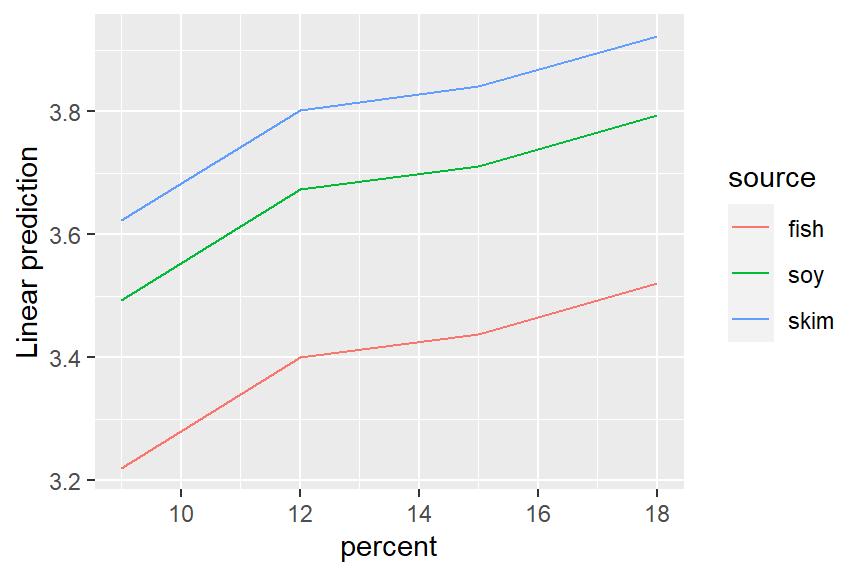emmip(ref_grid(pigs.lm2, cov.reduce = FALSE), source ~ percent)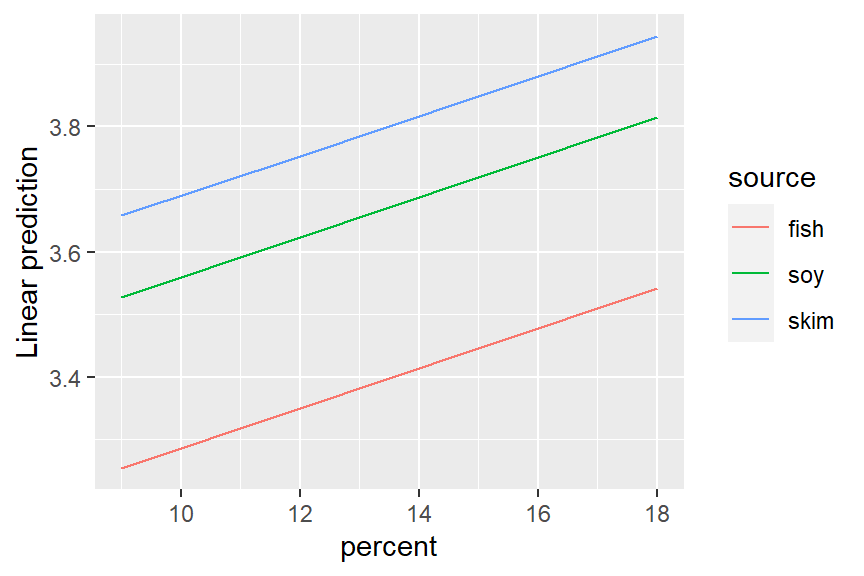Notice that emmip() may also be used on a fitted model. The formula specification needs the x variable on the right-hand side and the “trace” factor (what is used to define the different curves) on the left. This is a good time to yet again emphasize that EMMs are based on a model. Neither of these plots is an interaction plot of the data; they are interaction plots of model predictions; and since both models do not include an interaction, no interaction at all is evident in the plots.

The other graphics option offered is the plot() method for emmGrid objects. In the following, we display the estimates and 95% confidence intervals for mtcars.rg in separate panels for each disp.

plot(mtcars.rg, by = "disp")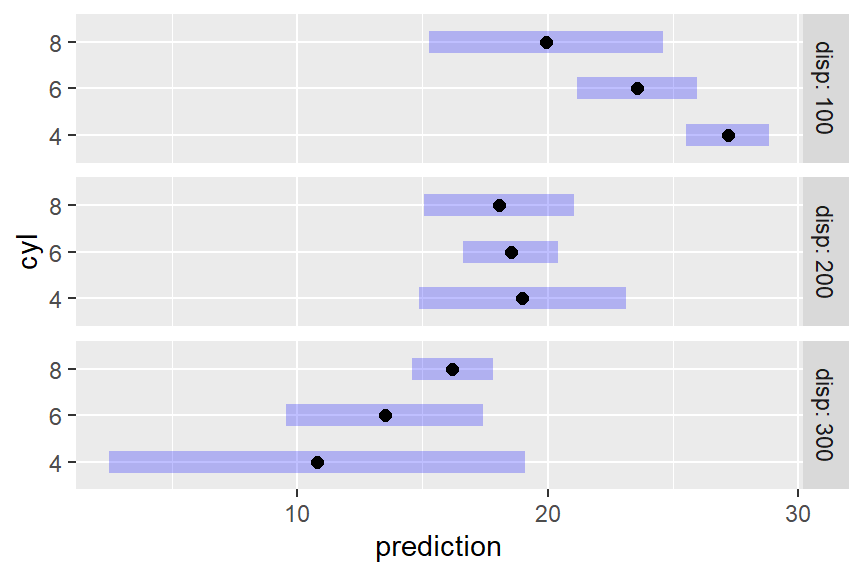This plot illustrates, as much as anything else, how silly it is to try to predict mileage for a 4-cylinder car having high displacement, or an 8-cylinder car having low displacement. The widths of the intervals give us a clue that we are extrapolating. A better idea is to acknowledge that displacement largely depends on the number of cylinders. So here is yet another way to use cov.reduce to modify the reference grid:

mtcars.rg_d.c <- ref_grid(mtcars.lm, at = list(cyl = c(4,6,8)),
cov.reduce = disp ~ cyl)
mtcars.rg_d.c @ grid
##        disp cyl .wgt.
## 1  93.78673   4     1
## 2 218.98458   6     1
## 3 344.18243   8     1

The ref_grid call specifies that disp depends on cyl; so a linear model is fitted with the given formula and its fitted values are used as the disp values – only one for each cyl. If we plot this grid, the results are sensible, reflecting what the model predicts for typical cars with each number of cylinders:

plot(mtcars.rg_d.c)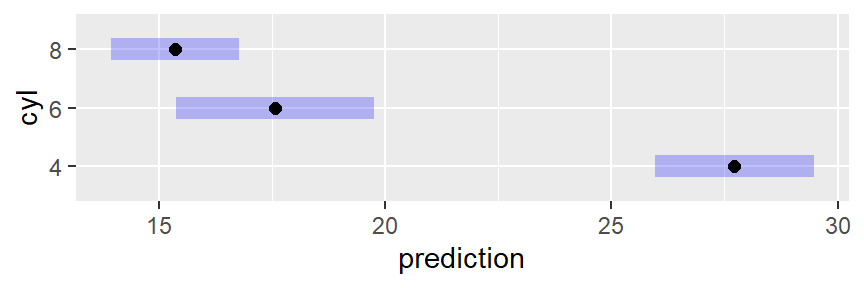Wizards with the ggplot2 package can further enhance these plots if they like. For example, we can add the data to an interaction plot – this time we opt to include confidence intervals and put the three sources in separate panels:

require("ggplot2")
## Loading required package: ggplot2
emmip(pigs.lm1, ~ percent | source, CIs = TRUE) +
geom_point(aes(x = percent, y = log(conc)), data = pigs, pch = 2, color = "blue")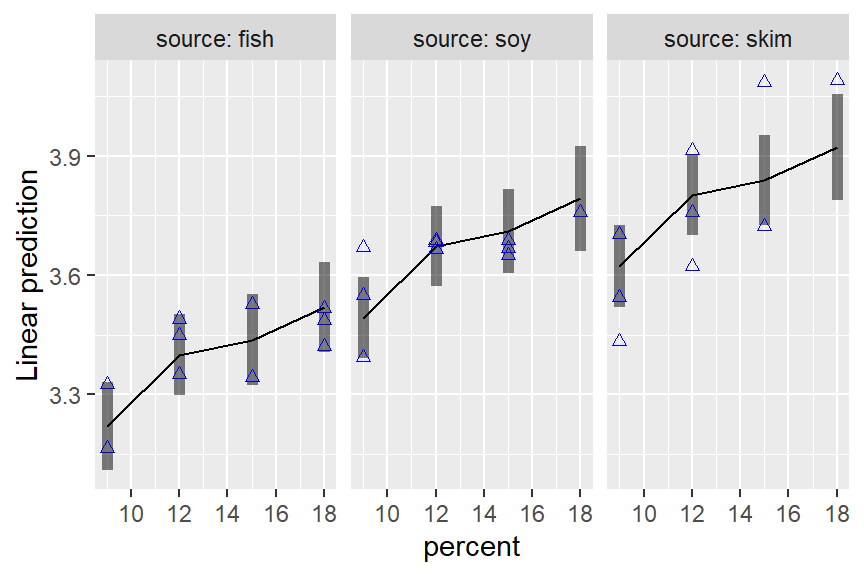Back to Contents

### Using weights

It is possible to override the equal-weighting method for computing EMMs. Using weights = "cells" in the call will weight the predictions according to their cell frequencies (recall this information is retained in the reference grid). This produces results comparable to ordinary marginal means:

emmeans(pigs.lm1, "percent", weights = "cells")
##  percent emmean     SE df lower.CL upper.CL
##        9   3.47 0.0407 23     3.39     3.56
##       12   3.62 0.0384 23     3.55     3.70
##       15   3.67 0.0435 23     3.58     3.76
##       18   3.66 0.0515 23     3.55     3.76
##
## Results are averaged over the levels of: source
## Results are given on the log (not the response) scale.
## Confidence level used: 0.95

Note that, as in the ordinary means in the motivating example, the highest estimate is for percent = 15 rather than percent = 18. It is interesting to compare this with the results for a model that includes only percent as a predictor.

pigs.lm3 <- lm(log(conc) ~ factor(percent), data = pigs)
emmeans(pigs.lm3, "percent")
##  percent emmean     SE df lower.CL upper.CL
##        9   3.47 0.0731 25     3.32     3.62
##       12   3.62 0.0689 25     3.48     3.77
##       15   3.67 0.0782 25     3.51     3.83
##       18   3.66 0.0925 25     3.46     3.85
##
## Results are given on the log (not the response) scale.
## Confidence level used: 0.95

The EMMs in these two tables are identical, but their standard errors are considerably different. That is because the model pigs.lm1 accounts for variations due to source. The lesson here is that it is possible to obtain statistics comparable to ordinary marginal means, while still accounting for variations due to the factors that are being averaged over.

Back to Contents

### Multivariate responses

The emmeans package supports various multivariate models. When there is a multivariate response, the dimensions of that response are treated as if they were levels of a factor. For example, the MOats dataset provided in the package has predictors Block and Variety, and a four-dimensional response yield giving yields observed with varying amounts of nitrogen added to the soil. Here is a model and reference grid:

MOats.lm <- lm (yield ~ Block + Variety, data = MOats)
ref_grid (MOats.lm, mult.name = "nitro")
## 'emmGrid' object with variables:
##     Block = VI, V, III, IV, II, I
##     Variety = Golden Rain, Marvellous, Victory
##     nitro = multivariate response levels: 0, 0.2, 0.4, 0.6

So, nitro is regarded as a factor having 4 levels corresponding to the 4 dimensions of yield. We can subsequently obtain EMMs for any of the factors Block, Variety, nitro, or combinations thereof. The argument mult.name = "nitro" is optional; if it had been excluded, the multivariate levels would have been named rep.meas.

Back to Contents

## Objects, structures, and methods

The ref_grid() and emmeans() functions are introduced previously. These functions, and a few related ones, return an object of class emmGrid:

pigs.rg <- ref_grid(pigs.lm1)
class(pigs.rg)
##  "emmGrid"
## attr(,"package")
##  "emmeans"
pigs.emm.s <- emmeans(pigs.rg, "source")
class(pigs.emm.s)
##  "emmGrid"
## attr(,"package")
##  "emmeans"

If you simply show these objects, you get different-looking results:

pigs.rg
## 'emmGrid' object with variables:
##     source = fish, soy, skim
##     percent =  9, 12, 15, 18
## Transformation: "log"
pigs.emm.s
##  source emmean     SE df lower.CL upper.CL
##  fish     3.39 0.0367 23     3.32     3.47
##  soy      3.67 0.0374 23     3.59     3.74
##  skim     3.80 0.0394 23     3.72     3.88
##
## Results are averaged over the levels of: percent
## Results are given on the log (not the response) scale.
## Confidence level used: 0.95

This is based on guessing what users most need to see when displaying the object. You can override these defaults; for example to just see a quick summary of what is there, do

str(pigs.emm.s)
## 'emmGrid' object with variables:
##     source = fish, soy, skim
## Transformation: "log"

The most important method for emmGrid objects is summary(). It is used as the print method for displaying an emmeans() result. For this reason, arguments for summary() may also be specified within most functions that produce these kinds of results.emmGrid objects. For example:

# equivalent to summary(emmeans(pigs.lm1, "percent"), level = 0.90, infer = TRUE))
emmeans(pigs.lm1, "percent", level = 0.90, infer = TRUE)
##  percent emmean     SE df lower.CL upper.CL t.ratio p.value
##        9   3.45 0.0409 23     3.38     3.52 84.262  <.0001
##       12   3.62 0.0384 23     3.56     3.69 94.456  <.0001
##       15   3.66 0.0437 23     3.59     3.74 83.757  <.0001
##       18   3.75 0.0530 23     3.65     3.84 70.716  <.0001
##
## Results are averaged over the levels of: source
## Results are given on the log (not the response) scale.
## Confidence level used: 0.9

This summary() method for emmGrid objects) actually produces a data.frame, but with extra bells and whistles:

class(summary(pigs.emm.s))
##  "summary_emm" "data.frame"

This can be useful to know because if you want to actually use emmeans() results in other computations, you should save its summary, and then you can access those results just like you would access data in a data frame. The emmGrid object itself is not so accessible. There is a print.summary_emm() function that is what actually produces the output you see above – a data frame with extra annotations.

Back to Contents

## P values, “significance”, and scientific integrity

There is a growing consensus among statisticians and researchers that P values are widely misunderstood, and that the term “statistical significance” is downright misleading. If you have a small P value, it only means that the effect being tested is unlikely to be explained by chance variation alone, in the context of the current study and the current statistical model underlying the test. If you have a large P value, it only means that the observed effect could plausibly be due to chance alone: it is wrong to conclude that there is no effect.

The American Statistical Association has for some time been advocating very cautious use of P values (Wasserman et al. 2014, 2019). The ASA now advises against ever using the term “statistically significant” (or a related descriptor) because it is too often misinterpreted, and too often used carelessly.

Wasserman et al. (2019), and the 43 articles it accompanies in the same issue of TAS, recommend a number of alternatives. I do not agree with all that is said in the main article, and there are portions that are too cutesy or wander off-topic. Further, it is quite dizzying to try to digest all the accompanying articles, and to reconcile their disagreeing viewpoints. But the basic ideas are summarized with the help of the acronym ATOM, for the four highlighted words below:

• Acceptance of uncertainty. If you were to repeat your study, you would get somewhat different results and perhaps different effects will stand out. Likewise, there are other reasonable models that you might have chosen, and those would have yielded different results. If the topic is worth studying, others will try to replicate it, perhaps with different study designs and different ways of operationalizing important concepts – again, yielding different results.

• Thoughtfulness. Design your study with clear objectives in mind, and spend time considering the best way to address those objectives in your study design. Pilot the study to ensure that the procedures actually work, how much variability to expect, and ensure that the sample size is adequate. How do your results fit in the big picture, scientifically? Think about the importance of the effects you have observed in your model(s)/data in the context of the science. Think carefully about how well your model fits the data and if there are other possibilities.

• Openness. Be transparent in disclosing the methods used, and reporting comprehensively all the successes and failures, the small and large effects. If hypothesis tests are used, report the actual P values found (e.g., “P = 0.075”) and don’t suppress or withhold results just because they are “nonsignificant,” because those results may still be useful to other researchers via meta-analysis. Trust in the expert judgment of other researchers.

• Modesty. Be clear in examining and expressing the limitations of your work. Understand that other researchers with different subject-area strengths may choose other models that may cast a different light on your work. Encourage others to try to reproduce your work, and be a good scientific colleague to them. Do not exaggerate the importance of your findings. Your study is not final or definitive; recognize that it is just one part of a broader picture. Ironically, the more important the research, the more related research will be undertaken, making that picture bigger (and, potentially, your part of it smaller).